Lab Week 12: Sorting Networks

Outline

1. Simple Sorting Algorithms
2. Sorting Networks
3. Sorting Activity

Last Time

Sorting by Divide and Conquer

• Merge sort
1. sort left half
2. sort left half
3. merge sorted halves
• Quicksort
1. pick pivot
2. split according to pivot
• left half less than pivot
• right half at least pivot
3. sort left half
4. sort right half

Sorting Networks

Another view of sorting in parallel

Insertion Sort, Revisited

for (int i = 1; i < data.length; ++i) {
for (int j = i; j > 0; --j) {
if (data[j-1] > data[j]) {
swap(data, j-1, j)
}
}
}

Appealing Features of Insertion Sort

for (int i = 1; i < data.length; ++i) {
for (int j = i; j > 0; --j) {
if (data[j-1] > data[j]) {
swap(data, j-1, j)
}
}
}
1. Only modifications are (adjacent) swaps
• sorting is in place
2. Access pattern is independent of input
• inputs always read/compared in same order
• only difference between execution is outcomes of swaps

Comparators: Visualizing Swaps

if (data[i] > data[j]) {
swap(data, i, j)
}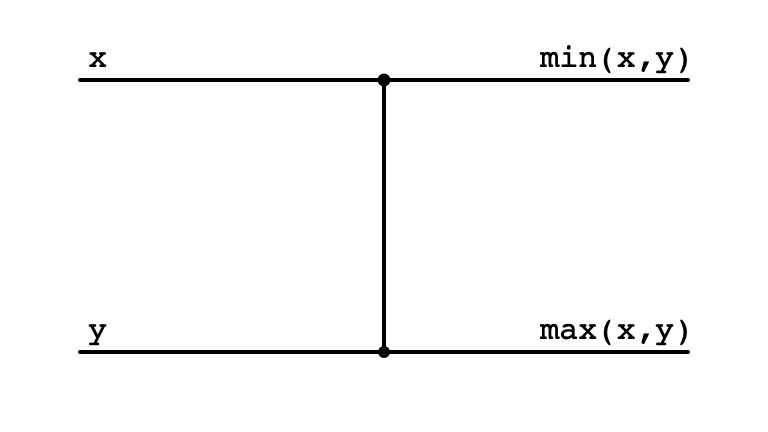Comparator Swap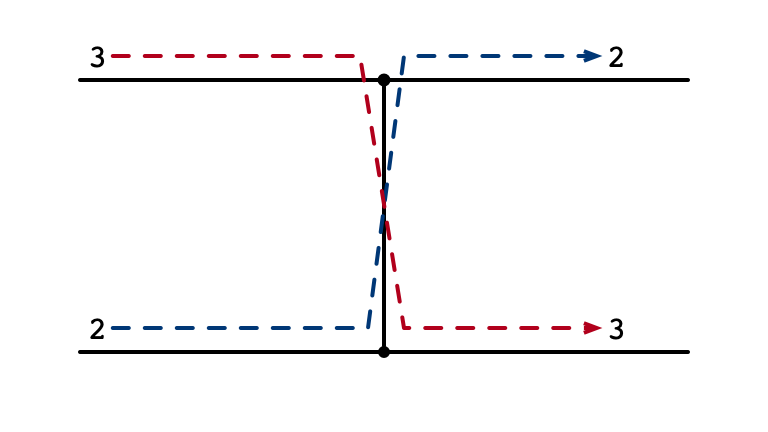Comparator No Swap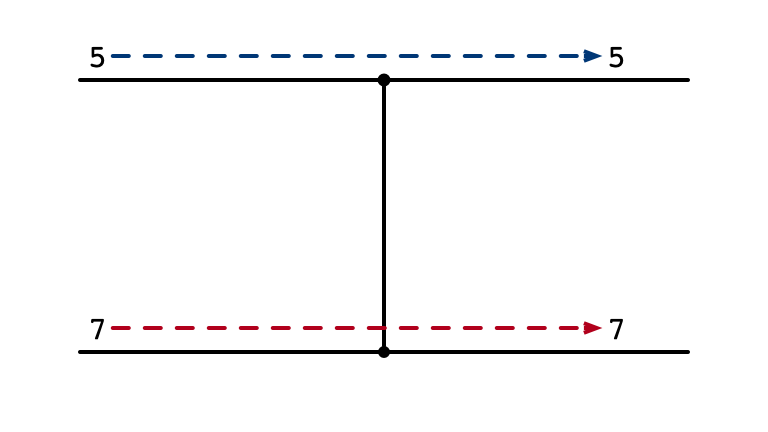Sorting Array of Two Elements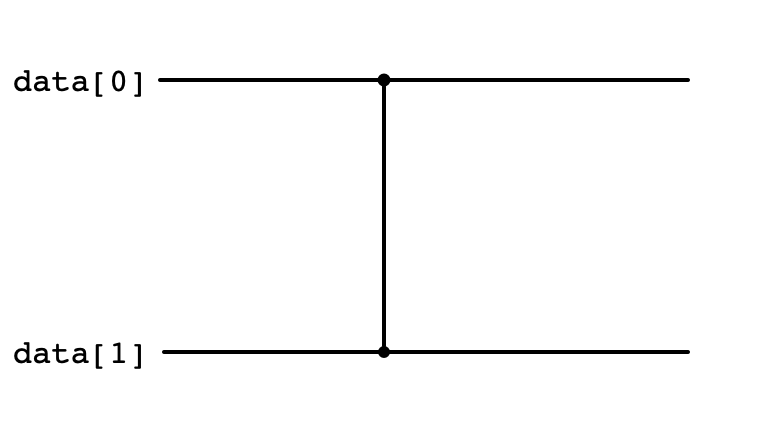Insertion Sort

for (int i = 1; i < data.length; ++i) {
for (int j = i; j > 0; --j) {
if (data[j-1] > data[j]) {
swap(data, j-1, j)
}
}
}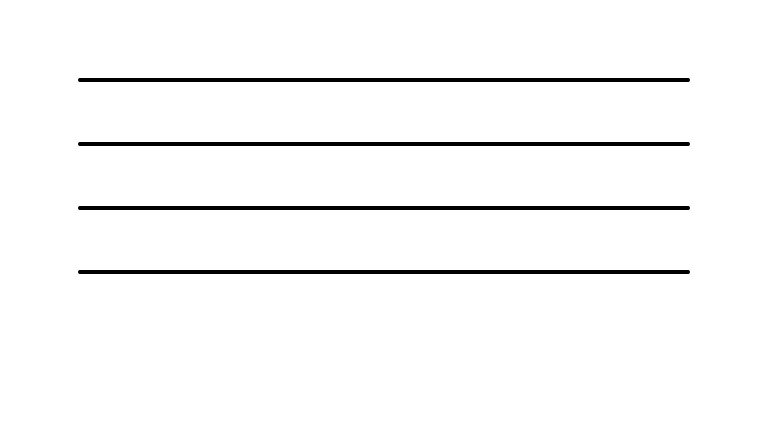Insertion Sort: i = 1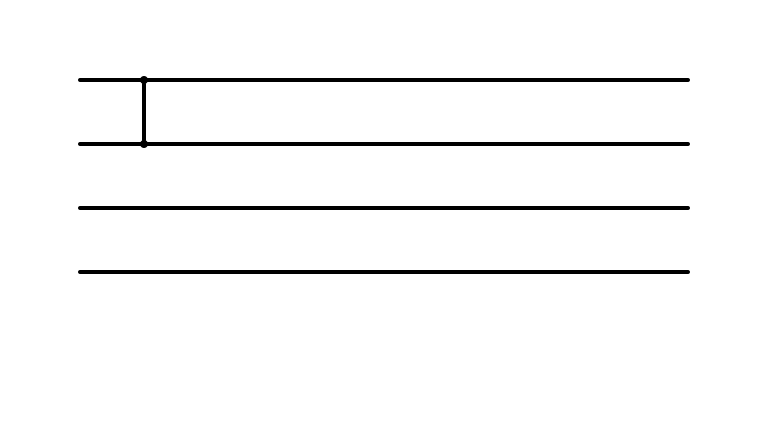Insertion Sort: i = 2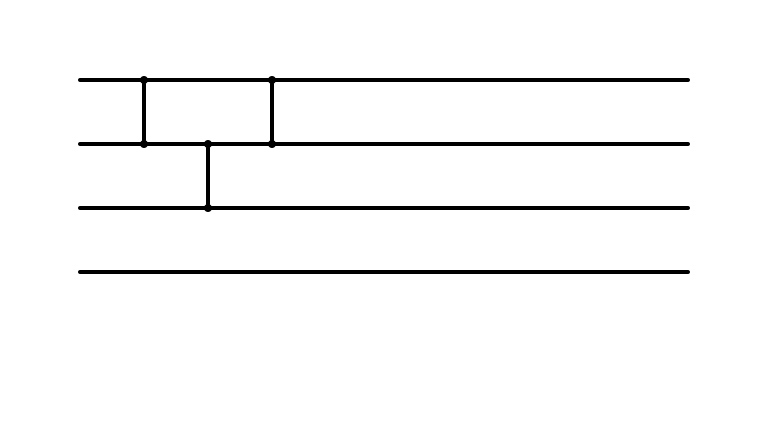Insertion Sort: i = 3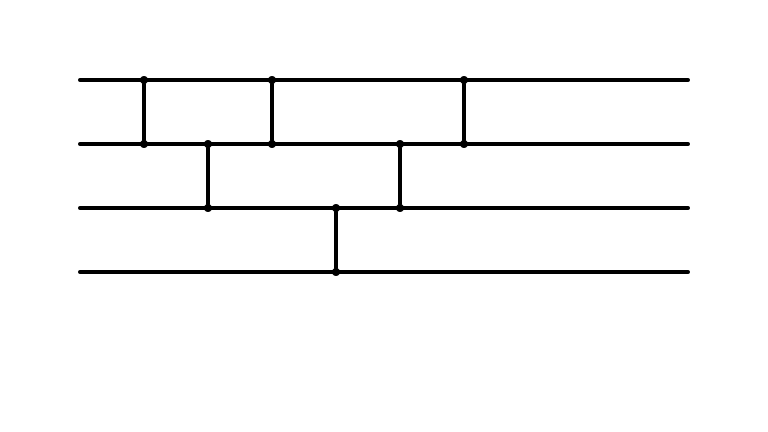Which Operations can be Parallelized?Parallelism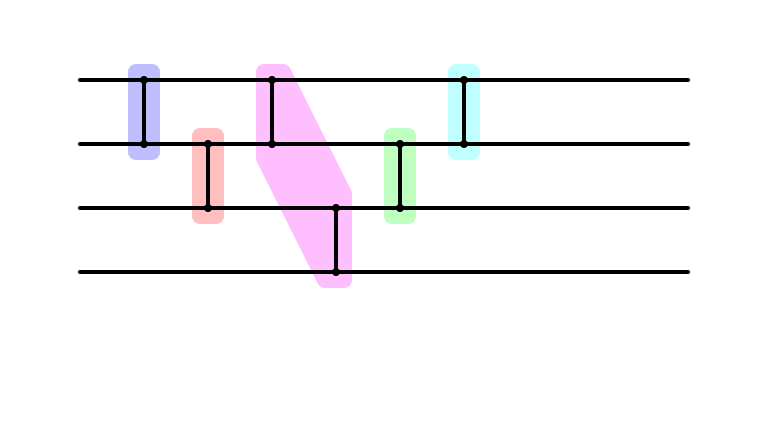Cleaner Parallel Representation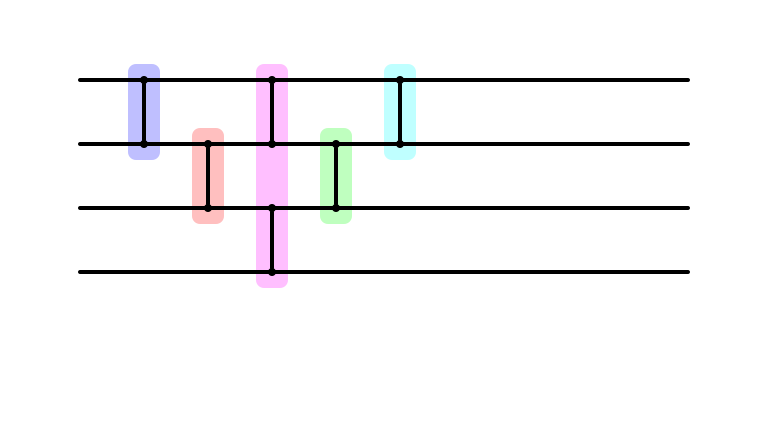Depth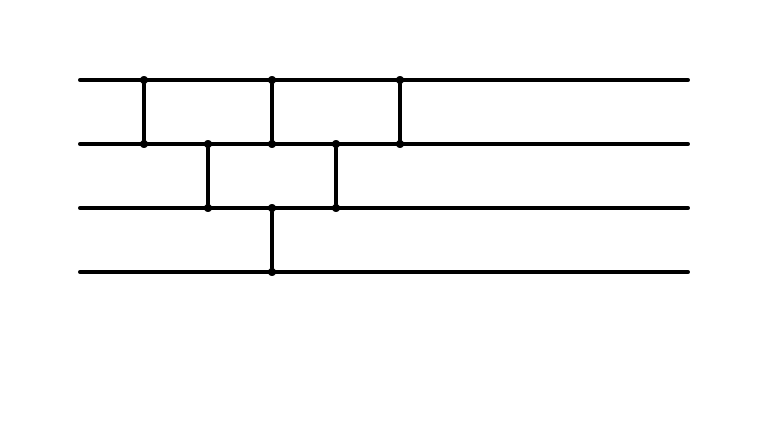Insertion Sort: Larger Instance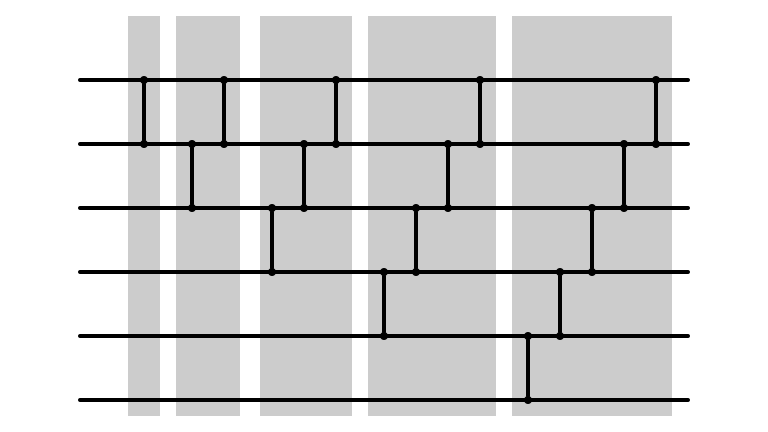Done In Parallel?Done In Parallel!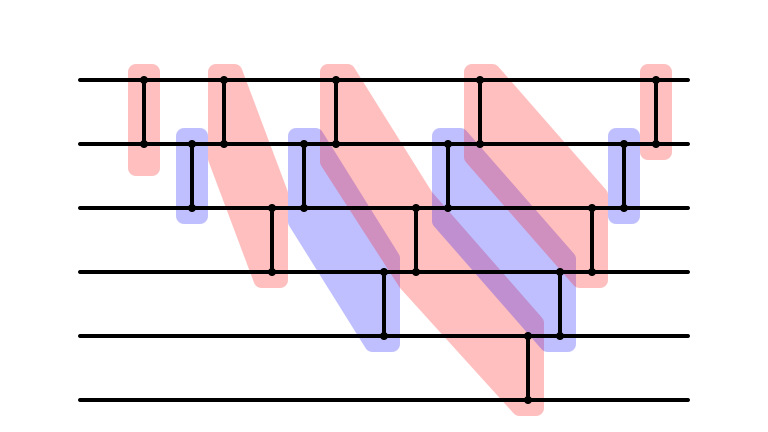Cleaned Up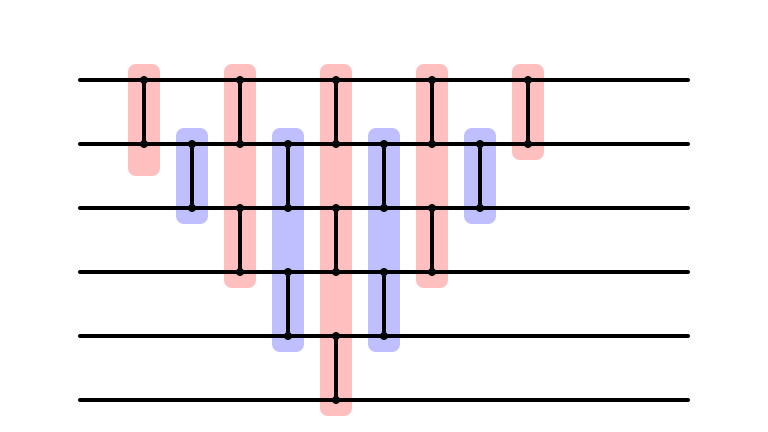Parallel Depth?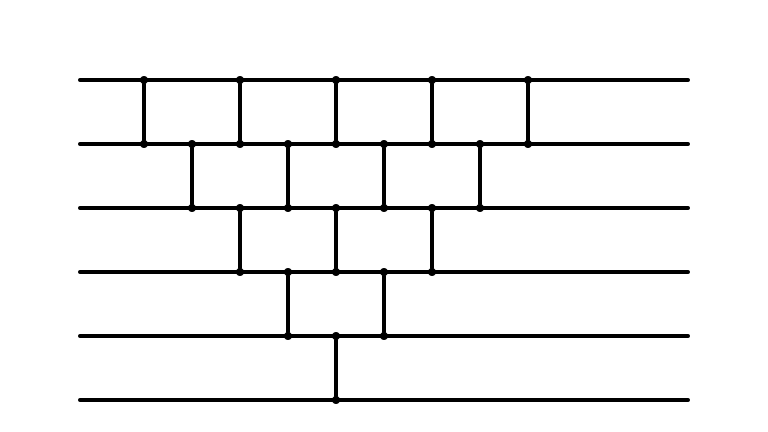Bubble Sort

Consider:

for (int m = data.length - 1; m > 0; --m) {
for (int i = 0; i < m; ++i) {
if (data[i] > data[i+1]) {
swap(data, i, i+1)
}
}
}

Can we make a sorting network corresponding to bubble sort?

Bubble Sort: m = 6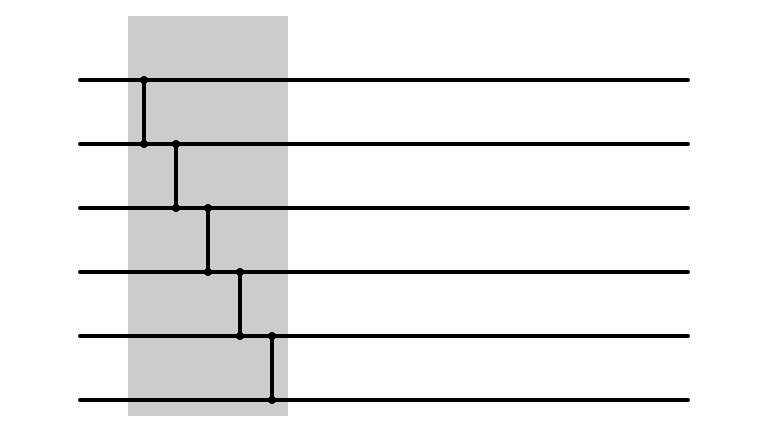Bubble Sort: m = 5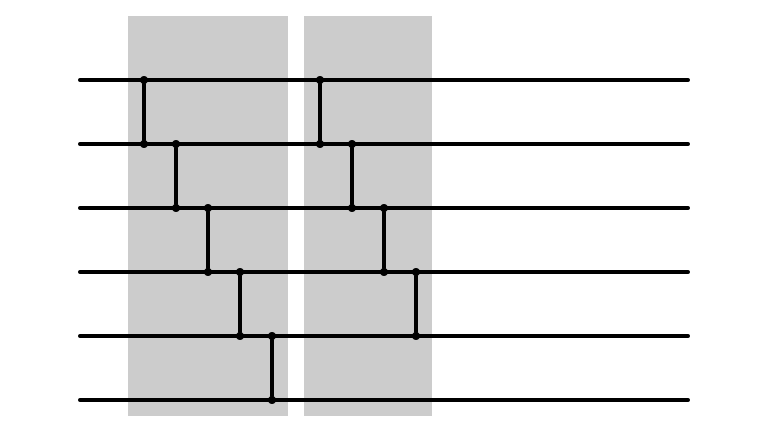Bubble Sort: m = 4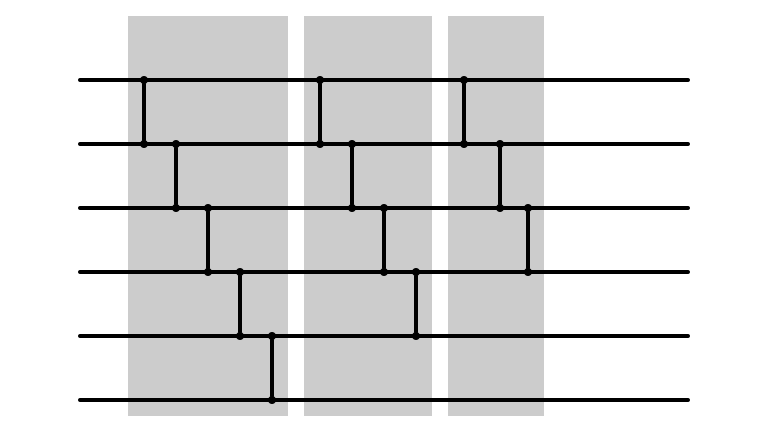Bubble Sort: m = 3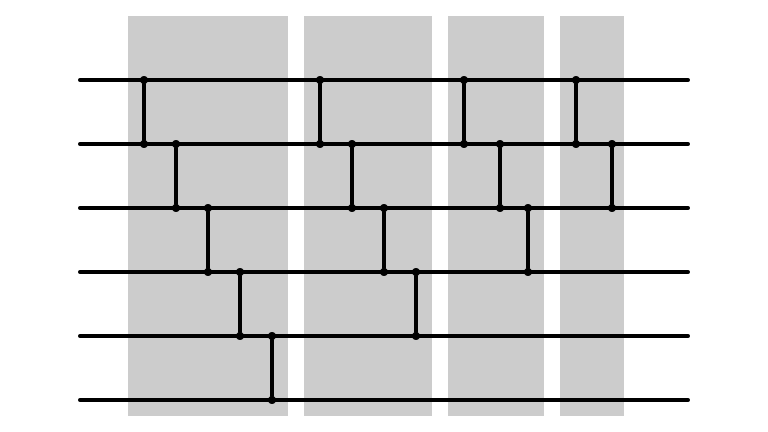Bubble Sort: m = 2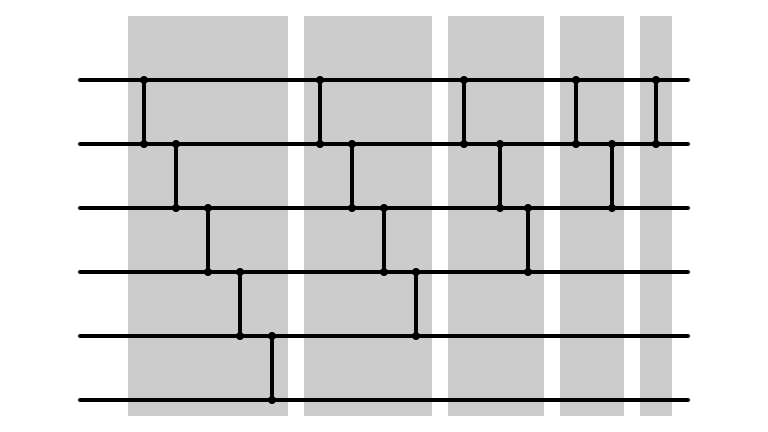Bubble Sort Network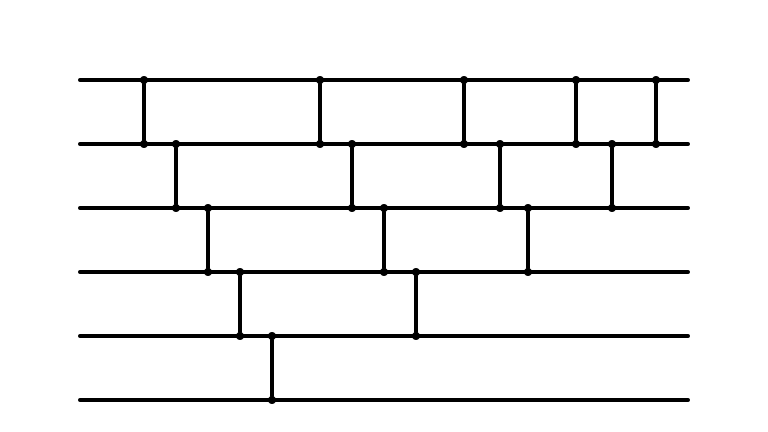Bubble Sort Parallelized?Does it Look Familiar?Huh

• Insertion sort and bubble sort perform precisely same operations
• only differ in the order in which comparisons are made
• When fuly parallelized, both are same sorting network

• Parallel versions are reasonably efficient
• depth $2 (n - 1) - 1 = 2 n - 3$

Applications

• Sorting networks can be efficiently implemented in hardware
• can sort fixed number of items much faster than software sorting
• Depth corresponds to latency
• smaller depth = faster computations

Activity

Find a minimum depth sorting networks for small $n$!

Current State

What is known:

• Optimal depth sorting networks for $n \leq 17$

What is not known:

• Optimal depth sorting networks for $n \geq 18$